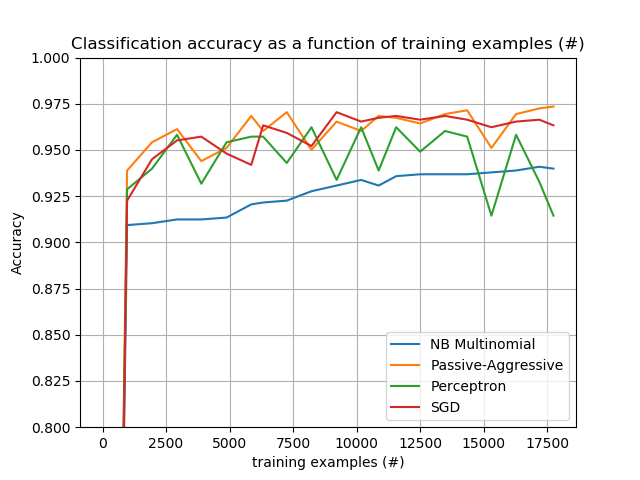# sklearn.feature_extraction.HashingVectorizer¶

class sklearn.feature_extraction.text.HashingVectorizer(*, input='content', encoding='utf-8', decode_error='strict', strip_accents=None, lowercase=True, preprocessor=None, tokenizer=None, stop_words=None, token_pattern='(?u)\b\w\w+\b', ngram_range=(1, 1), analyzer='word', n_features=1048576, binary=False, norm='l2', alternate_sign=True, dtype=<class 'numpy.float64'>)

[源码]

• 它的内存非常低，可扩展到大型数据集，因为不需要在内存中存储词汇字典
• 它可以快速地pickle和解pickle，因为除了构造函数参数之外，它不包含任何状态
• 它可以在流(部分fit)或并行管道中使用，因为在fit期间没有计算状态。

• 没有办法计算逆变换(从特征索引到字符串特征名称)，这可能是一个问题，当试图内省哪些特征是最重要的模型。
• 可能会有冲突:不同的令牌可以映射到相同的特性索引。然而，在实践中，如果n_features足够大(例如，文本分类问题是2 ** 18)，这很少会成为一个问题。
• 没有IDF权重，因为这会使转换器有状态。

input string {‘filename’, ‘file’, ‘content’}, default=’content’

encodingstring默认=“utf - 8”

encoding string, default=’utf-8’

decode_error {‘strict’, ‘ignore’, ‘replace’}, default=’strict’

strip_accents {‘ascii’, ‘unicode’}, default=None

' ascii '和' unicode '都使用NFKD标准化从unicodedata.normalize。
lowercase bool, default=True

preprocessor callable, default=None

tokenizer callable, default=None

stop_words string {‘english’}, list, default=None

token_pattern string

ngram_range tuple (min_n, max_n), default=(1, 1)

analyzer string, {‘word’, ‘char’, ‘char_wb’} or callable, default=’word’

n_features int, default=(2 \ 20)

binary bool, default=False.

norm {‘l1’, ‘l2’}, default=’l2’

alternate_sign bool, default=True

dtype type, default=np.float64
fit_transform()transform()返回的矩阵的类型。

>>> from sklearn.feature_extraction.text import HashingVectorizer>>> corpus = [...     'This is the first document.',...     'This document is the second document.',...     'And this is the third one.',...     'Is this the first document?',... ]>>> vectorizer = HashingVectorizer(n_features=2**4)>>> X = vectorizer.fit_transform(corpus)>>> print(X.shape)(4, 16)

build_analyzer() 返回处理预处理、记号化和生成n个符号的可调用函数。
build_preprocessor() 返回一个函数，用于在标记之前对文本进行预处理。
build_tokenizer() 返回一个函数，该函数将字符串分割为一系列标记。
decode(doc) 将输入解码为unicode符号字符串。
fit(X[, y]) 什么也不做:这个转换器是无状态的。
fit_transform(X[, y]) 将文档序列转换为文档术语矩阵。
get_params([deep]) 获取这个估计器的参数。
get_stop_words() 构建或获取有效停止词列表。
partial_fit(X[, y]) 什么也不做:这个转换器是无状态的。
set_params(**params) 设置的参数估计量。
transform(X) 将文档序列转换为文档术语矩阵。
__init__(*, input='content', encoding='utf-8', decode_error='strict', strip_accents=None, lowercase=True, preprocessor=None, tokenizer=None, stop_words=None, token_pattern='(?u)\b\w\w+\b', ngram_range=(1, 1), analyzer='word', n_features=1048576, binary=False, norm='l2', alternate_sign=True, dtype=<class 'numpy.float64'>)

[源码]

build_analyzer() 

[源码]

analyzer callable

build_preprocessor()

[源码]

preprocessor callable

build_tokenizer()

[源码]

tokenizer callable

decode(doc)

[源码]

doc str

doc str

fit(X, y=None)[source]

[源码]

X ndarray of shape [n_samples, n_features]

fit_transform(X, y=None)

[源码]

X iterable over raw text documents, length = n_samples

y any

X sparse matrix of shape (n_samples, n_features)
Document-term矩阵。
get_stop_words()

[源码]

stop_words list or None

partial_fit(X, y=None)

[源码]

X ndarray of shape [n_samples, n_features]

set_params(**params)

[源码]

**params dict

self object

transform(X)

[源码]

X iterable over raw text documents, length = n_samples

X sparse matrix of shape (n_samples, n_features)
Document-term矩阵。

## 示例sklearn.feature_extraction.text.HashingVectorizer¶